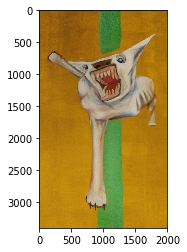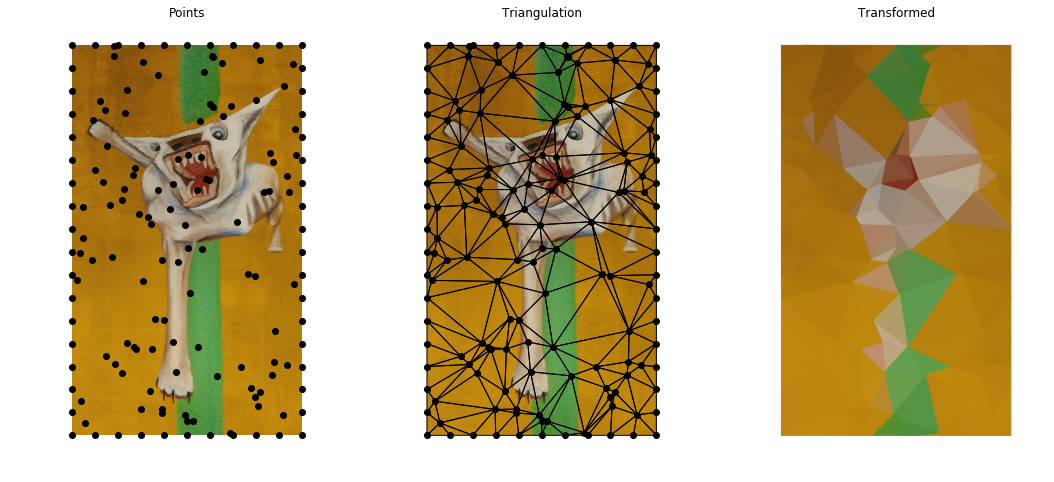# Images to Triangles

Published: 24/05/2017

In the last few days I came across this stack exchange code-golf challenge about reducing a photo to a set of polygons using a Voronoi diagram. I'm going to have a go, but I'm going to change the goal a bit. Rather then creating polygons, I'm going to use a Delaunay triangulation to turn an image into a set of triangles.

My reason for approaching the problem like this is that Voronoi maps create polygons which are centered around points. In a triangulation, the points are the vertices. This means that if we can identify the points of an image which match the natural boundaries, the vertices of our triangulation should follow them. The question is how we identify what constitutes an "interesting" point.

Before we get into that, some notes on:

• A Triangulation of a set of points is a way of covering the convex hull defined by the points with triangles (or simplices in higher dimensions) such that the points are the vertices of the triangles and any two triangles either share a complete edge, or do not intersect at all.
• There are many ways a set of points can be split into a triangulation
• The Delaunay triangulation is defined as a triangulation such that each circumcircle of the triangles does not contain any points. This has the property that is maximises the minimum angle - making the triangles appear even and not unnecessarily sharp. It is this property that makes it appealing to use for subdividing an image.

The notes here have a good discussion of Delaunay triangulation and how it is calculated. We will be using the scipy implementation.

As with my previous post on image processing, I'm going to use the following image

In :
import numpy as np
import matplotlib.pylab as plt
from scipy.spatial import Delaunay

%matplotlib inline

im = im[400:3800,:2000,:]
plt.imshow(im)

Out:
<matplotlib.image.AxesImage at 0x7f82900a54a8># Uniform Random Points¶

Let's start with the simplest approach. We will generate $N$ points uniformly over the image and look at the resulting patterns formed by applying the triangulation.

Most of the code here is wrapped up in a utility module "triangulared". The full code for both this module, and the notebook that produces this post can be found here.

It applies the following steps:

• Generate a set of points for an image
• Generates the triangulation of these points
• Replaces the image with a set of triangles coloured with the median value of the pixels they cover

The results are below

In :
from triangulared import *

In :
points = generate_uniform_random_points(im, n_points=100)
tri = Delaunay(points)

fig, axs = plt.subplots(ncols=3, figsize=(18,8), sharey=True)

ax = axs
draw_image(ax, im)
draw_points(ax, points)
ax.set_title("Points")
set_axis_defaults(ax)

ax = axs
draw_image(ax, im)
draw_points(ax, points)
draw_triangles(ax, tri.points, tri.vertices)
ax.set_title("Triangulation")
set_axis_defaults(ax)

ax = axs
triangle_colours = get_triangle_colour(tri, im)
draw_triangles(ax, tri.points, tri.vertices, triangle_colours)
ax.set_title("Transformed")
set_axis_defaults(ax)If we know the original image, we might be able to guess what the transformed image is, but it is not clear. Things get a bit better when we increase the number of points:

In :
fig, axs = plt.subplots(ncols=3, figsize=(18,8), sharey=True)

for ax, n_points in zip(axs, [500,1000,5000]):
points = generate_uniform_random_points(im, n_points=n_points)
tri = Delaunay(points)
ax.invert_yaxis()
triangle_colours = get_triangle_colour(tri, im)
draw_triangles(ax, tri.points, tri.vertices, triangle_colours)
ax.set_title("n_points = {}".format(n_points))
set_axis_defaults(ax)